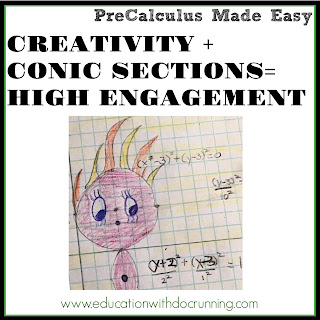Analytic geometry (a.k.a. conic sections) used to be my least favorite unit to teach.  The formulas are dry and easy to mix up, it's hard to find real world applications where my students might need these equations (or how to find the directrix, vertex, etc.), and they almost seem like a side topic from all the other work we have been doing in PreCalculus.  When I find a topic dull, I take it as a personal challenge to make it interesting to my students.  Over the past two years, I have fallen in love with conic sections.  Here's what I do to keep it interesting:

1. Start with the distance and midpoint formulas: Why?  Although students have seen these in Geometry, the distance and midpoint formula are directly related to parts of conic sections.   For example, the radius can be found using the midpoint formula if you have the diameter of a circle.  It's so obvious, but a part of analytic geometry that is often skipped.2. Making the formulae meaningful:  I stumbled upon this derivation of conic sections which was fascinating.  So much so that I made it into a short video for my students.  I start with it because it adds meaning.  We also create clay conic sections if possible.  Yes, in high school!  I divide students into groups of four with each student taking a different conic section to create.  I give them each a ball of self-drying clay (you can also just use play-doh purchase or homemade).  Students make cuts with string according to diagrams I give out on cards.  (You can also have each student create all 4 as shown on hollymath).  If you want more direction, here's a video on making conic sections from clay or if you have longer, check out Jeremy York's explanation here.3. Words, equations and diagrams: Next step is to put the shapes into words, equations and diagrams.  We use color graphic organizers to put each of the conic sections (parabolas, circles, ellipses, and hyperbolas) into words, equations and diagrams.  The vertex in each diagram is represented by the same color to help students make the connections between the different conic sections.4. Creative assessment (with a little fun):  When there is ready access to the equations for all the conic sections, I find little value in just giving students a test.  It is more important to me that they apply the formulas and get to be a little creative.  I have 2 projects that I give students.  The first, for hyperbolas, I use instead of straight practice problems.  Students look at hyperbolas being used in architecture and develop equations for the hyperbolas.  Then, students design a building in a hyperbolic shape and find the equation as well as vertices, directrix, etc.  At the end of the unit, students complete an open-ended project in which students created a themed image that includes all four conic sections with relevant information.  This is by far one of my students' favorite activities we do all semester.

And all of this came out of my personal quest to make a topic that I personally found dull a little more interesting.  Next challenge...limits!

This is part of a series of posts focused on teaching PreCalculus.  Topics include:

PreCalculus Day 1
Functions and Graphs
Polynomials
Exponents and Logarithms
Trigonometric Functions
Applications of Trigonometry
Systems of Equations and Matrices
Analytic Geometry in 2 and 3 dimensions
Discrete Mathematics
Introducing Limits - the Calculus of PreCalculus

Math Mondays is a bi-weekly blog post (2nd and 4th Monday of each month) sharing tips, ideas, resources, and products for teaching math.  If you have questions or think there is something I should include, you can leave me a message in the comments section below or at the store in the question and answer section.